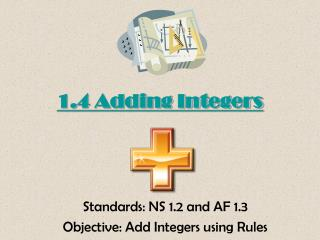DownloadDownload PresentationDownload Presentation- - - - - - - - - - - - - - - - - - - - - - - - - - - E N D - - - - - - - - - - - - - - - - - - - - - - - - - - -
Presentation Transcript

1. 1.4 Adding Integers Standards: NS 1.2 and AF 1.3 Objective: Add Integers using Rules

2. -7 -6 -5 -4 -3 -2 -1 0 1 2 3 4 5 6 Adding Integers • Use the number line to find the sum. Example: -3 + (-5) -3 + -5 = -8 • - - + - - - - - = -8

3. -7 -6 -5 -4 -3 -2 -1 0 1 2 3 4 5 6 Adding Integers • Use the number line to find the sum. Example: -9 + 6 • - - - - - - - - -9 + + + + + + + 6 -3

4. -7 -6 -5 -4 -3 -2 -1 0 1 2 3 4 5 6 Adding Integers Use the + and – to show the work. • -4 + (-6) • 4 + (-6) • -4 + 6

5. Adding Integers We are going to predict patterns and make rules based on what we notice. Then, we are going to simplify variable expressions.

6. Adding Integers Solve the problems by using + and – to show your work. • 4 + 5 • 16 + 13 Predict: What do you notice about your answers? What rule can we make?

7. Adding Integers Rule 1: Adding positives will give you a positive. + + + = +

8. Adding Integers Solve the problems by using + and – to show your work. • -12 + (-3) • -5 + (-17) Predict: What do you notice about your answers? What rule can we make?

9. Adding Integers Rule 2: Adding negatives will give you a negative. - + - = -

10. Adding Integers Solve the problems by using + and – to show your work. • -5 + 8 • -6 + 13 • 10 + (-7) Predict: What do you notice about your answers? What rule can we make?

11. Adding Integers Rule 3: Adding a BIGGER positive and smaller negative will give you a positive. TAKE THE SIGN OF THE BIGGER NUMBER. ++-=+

12. Adding Integers Solve the problems by using + and – to show your work. • -4 + 1 • -11 + 3 • 12 + (-17) Predict: What do you notice about your answers? What rule can we make?

13. Adding Integers Rule 4: Adding a smaller positive and BIGGER negative will give you a NEGATIVE. TAKE THE SIGN OF THE BIGGER NUMBER. ++-=-

14. Adding Integers Solve. • -9 + 14 • -7 + -2 • 4 + (-11) • 6 + (-2) + 3 + (-8)

15. Simplify Expressions Simplify the expressions by combining like terms. • 4n + (-6n) + 3 • 21w + (-17w) + 6w • 5x + (-2x) + 7

16. Evaluate Expressions Evaluate (solve) by combining like terms and substituting. • -5x + 14x + (-6x) when x = 2 • 8x + (-7) + (-5x) when x = 2

17. Adding Integers You can use integer addition in real-life situations when you know the direction and the amount of change in a quantity.

18. Adding Integers 1) The temperature at 4 pm Tuesday was 14° Fahrenheit. By 6 am Wednesday, the temperature had dropped 21°. What was the temperature?